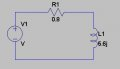# Calculating complex power (VA) on a sinus input.

#### supermankid

Joined May 26, 2013
54
Hi all,
I am trying to find the complex power(apparent power) of the circuit with input 200Vrms. The input is applied to the equivalent resistance (resistor + inductor) (0.8+5.6j). The complex power is calculated as |V|^2/(0.8-5.6j)= 200^2/(0.8-5.6j).
I understand all the logic but how is the impedance (0.8+5.6j) changed to (0.8-5.6j) during the calculation. (normally, impedance for resistor in series with inductor = r+jwL) but why is -(minus) used during the calculation?#### Papabravo

Joined Feb 24, 2006
14,207
You missed something important. You did not just change the sign. Your expression for complex power is:

$$\frac{V^2}{Z}$$

$$\frac{V^2}{0.8 + 5.6j}$$

To simplify this expression we multiply both numerator an denominator by the complex conjugate of the impedance.

$$\frac{(V^2)(0.8\;-\;5.6j)}{(0.8\;+\;5.6j)(0.8\;-\;5.6j)}$$

$$\frac{(V^2)(0.8\;-\;5.6j)}{(0.64\;+\;31.36)}$$

$$\frac{(V^2)(0.8\;-\;5.6j)}{32}$$

$$\frac{(V^2)(Z^*)}{Z^2}$$

The factor of 32 comes from the product of the impedance and its complex conjugate

Last edited:
•#### Papabravo

Joined Feb 24, 2006
14,207
What is not to understand?
1. Complex Current is Voltage divided by Impedance
2. Complex Power is the squared magnitude of voltage divided the complex conjugate of Impedance
3. Complex Power is also the Complex Voltage times complex conjugate of Current
4. The total Complex Power is the sum the Complex Powers in each leg of a parallel circuit
5. The Complex Current is the sum of the Complex Currents in each leg
Lastly and most important, there is more than one way to get a result and the results you get with any method you choose have to be the same. One other point is that you must be fluent with the different representations of complex quantities, and be able to convert from the Cartesian Representation to the polar representation without batting an eyelash.

•supermankid